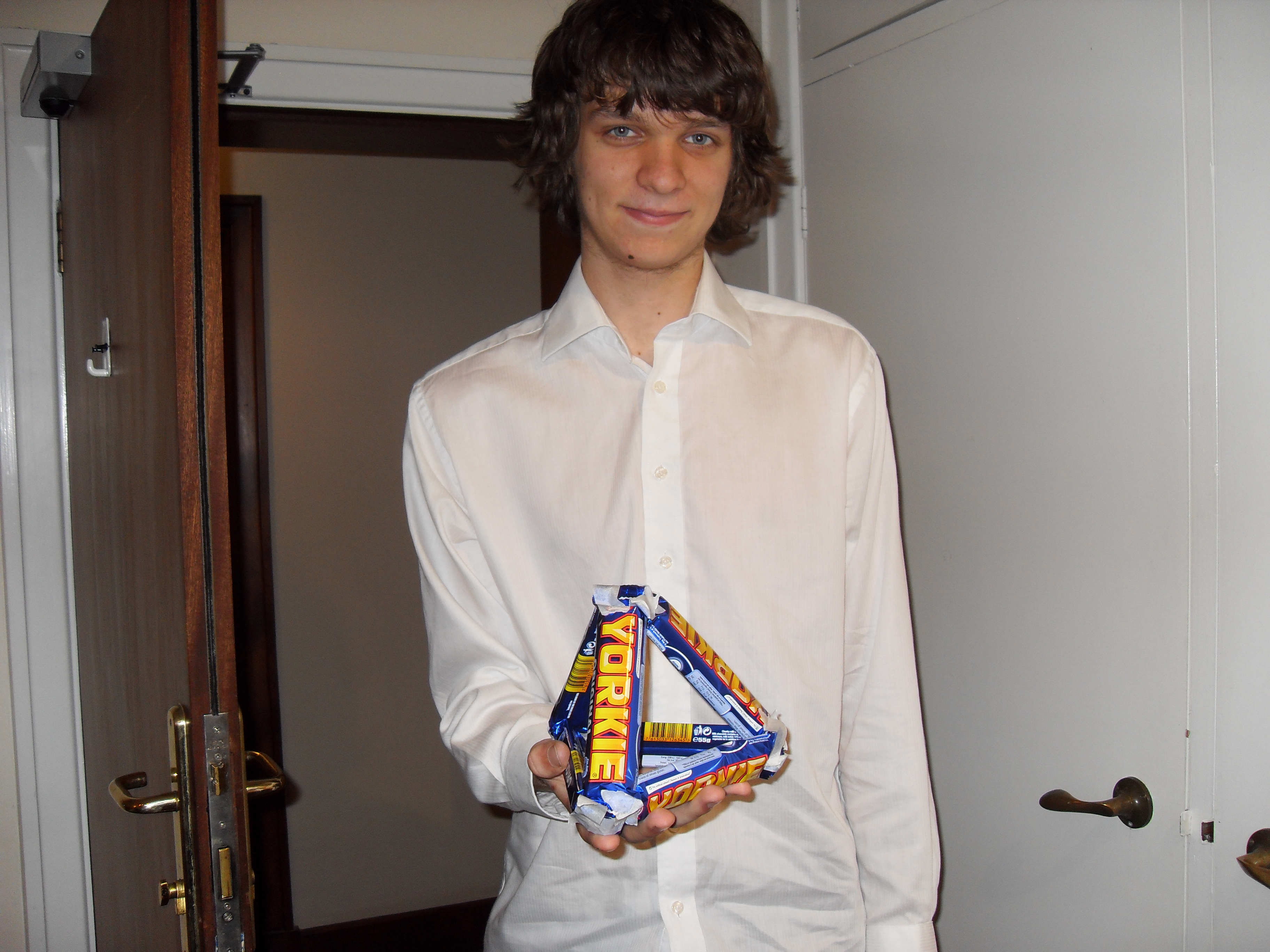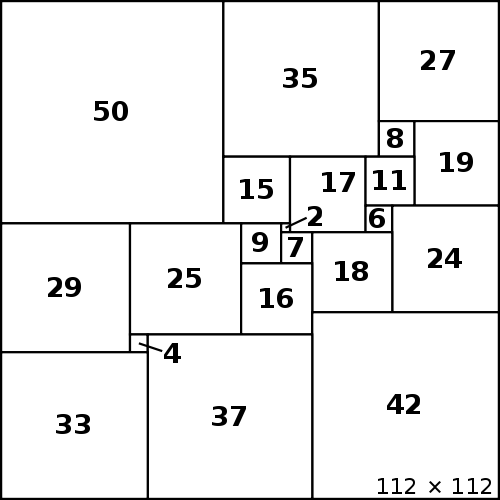# Miscellany

Tsukamoto and Miyazaki of Kyoto University have discovered that three states are sufficient to enable gliders to exist on a Penrose tiling, rather than four. The following video demonstrates their discovery together with a periodic emitter in an augmented rule:

On a related topic, my paper on the original Penrose gliders is now online. The derivation for sequence A215878 is included, which makes heavy use of Lindenmayer systems to describe the fractal paths traced out by the kite-and-dart gliders.

Suppose a Yorkie chocolate bar has a resistance of precisely one ohm. Can you determine the resistance between two vertices of the tetrahedron, which Rychlik is holding in the picture below? (Hint: Use the Y-Δ transform and formulae for resistors in series and parallel.)Yes, it’s 1/2 ohm. Similar analysis has been performed on the other Platonic solids, with the most complex being the dodecahedron (20 vertices and 30 edges). On a related topic, there was a challenge in the Google Labs Aptitude Test to determine the equivalent resistance between two points separated by a knight’s move on an infinite grid of ideal one-ohm resistors. Some complicated Fourier analysis gives the result as 4/π – 1/2, as does successive approximation followed by use of Robert Munafo’s Rilybot Inverse Equation Solver (a very useful tool for enabling one to dine with a girl whilst keeping her ex-boyfriend safely away by organising an [insert irrational constant]th birthday party for him!).Squared squares (such as the one above, the logo of the Trinity Mathematical Society) were discovered by considering networks of resistors. Place copper strips along the top and bottom of the square and apply a voltage between them. The resistance is unchanged when horizontal lines are replaced with copper strips and vertical lines are replaced with insulating strips. Then, the resistance across each square (including the large one) is one ohm. Consequently, this squared square corresponds to a network of one-ohm resistors with a total resistance of one ohm:The same argument applies to squared cylinders. Squared tori, on the other hand, are substantially easier to find: a 5×5 torus can be dissected into two squares of sizes 3×3 and 4×4, where {3,4,5} is a generic Pythagorean triple.

Finally, don’t forget that it’s Cipher Tuesday tomorrow. The cipher will be published at 00:01 UTC. I look forward to seeing you in the solvers’ area.

This entry was posted in Uncategorized. Bookmark the permalink.

### 0 Responses to Miscellany

1.Dan says:

Let’s not forget that a sqrt(5)xsqrt(5) torus can be tiled by five 1×1 squares.

•apgoucher says:

Indeed it can, but we’re generally interested in ‘perfect’ squared squares, where all of the squares are distinct integer sizes.

•Anonymous says:

twenty-five* 🙂

•Catisfluffy says:

No, because sqrt(5)^2=5.